Checkout JEE MAINS 2022 Question Paper Analysis : Checkout JEE MAINS 2022 Question Paper Analysis :

# Important Questions Maths Class 8 Chapter 4: Practical Geometry

Important questions for class 8 Maths Chapter 4 Practical Geometry are made available here for students to make them prepared for final exams 2020. These questions are available with solutions, provided by our subject experts to help them understand the concept well. Since the problems here are given based on CBSE(NCERT) latest syllabus, thus it will be easy for students to score good marks.

In this chapter, students of 8th standard will learn to construct the quadrilaterals based on certain given conditions such as: when the dimensions are given for its sides and diagonals or for angles. Let us see some of the important problems with their answers to have a proper revision here. Also, get Maths important questions for class 8 for all the chapters at BYJU’S.

## Important Questions With Solutions For Class 8th Maths Chapter 4 (Practical Geometry)

Q.1: Construct a quadrilateral ABCD where AB = 4.5 cm BC = 5.5 cm CD = 4 cm AD = 6 cm AC = 7 cm.

Solution: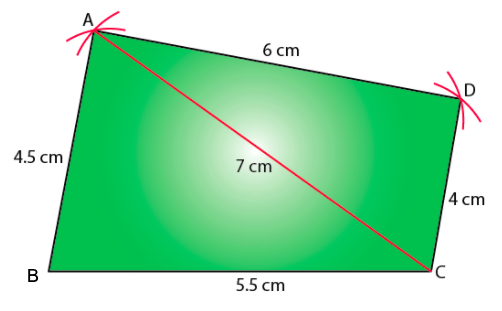Q.2: Construct a quadrilateral JUMP where JU = 3.5 cm UM = 4 cm MP = 5 cm PJ = 4.5 cm PU = 6.5 cm.

Solution: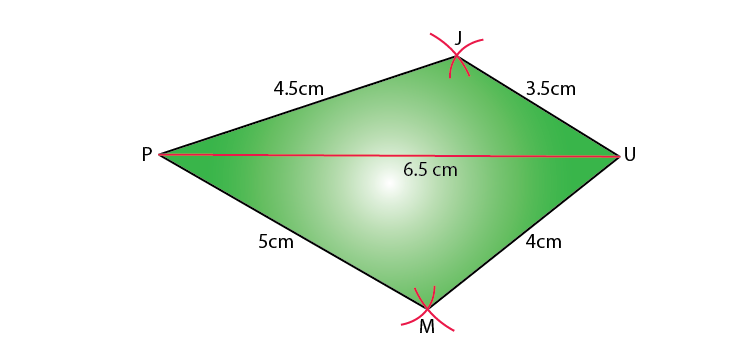Q.3: Construct a Rhombus BEND with BN = 5.6 cm DE = 6.5 cm.

Solution:

As we know, the diagonals of a rhombus bisect each other at a right angle.

Let us consider that these diagonals intersect each other at a point O in rhombus.

Hence, EO = OD = 3.25 cm

Here is a rough sketch of the rhombus BEND: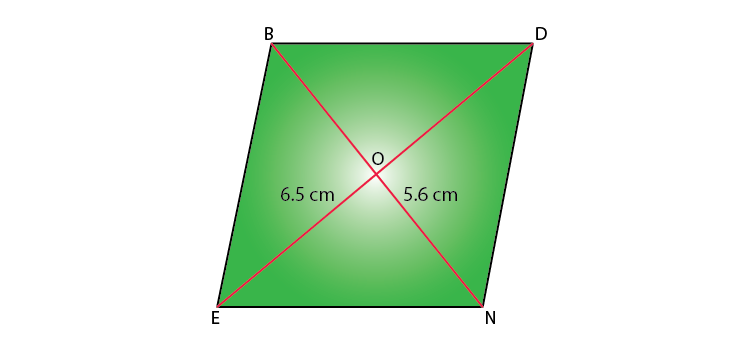Step 1: Draw a line segment BN of 5.6 cm and also draw its perpendicular bisector. It will intersect the line segment BN at point O.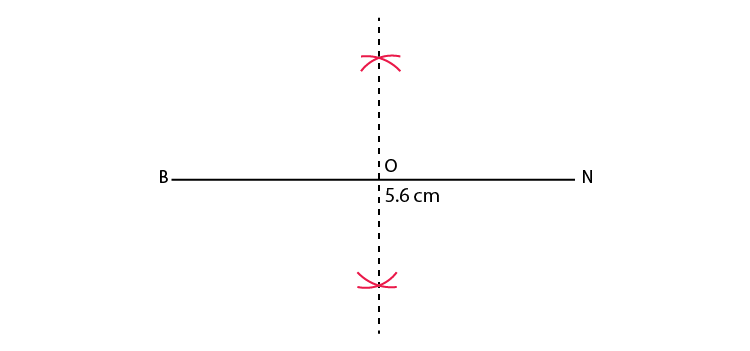Step 2: Taking O as the centre, draw arcs of 3.25 cm radius to intersect the perpendicular bisector at point D and E.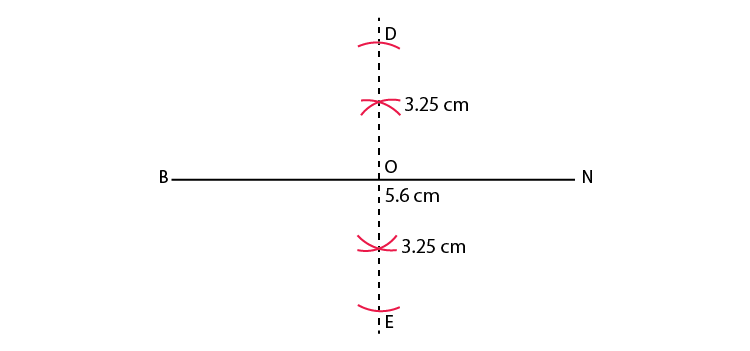Step 3: Join points D and E to points B and N.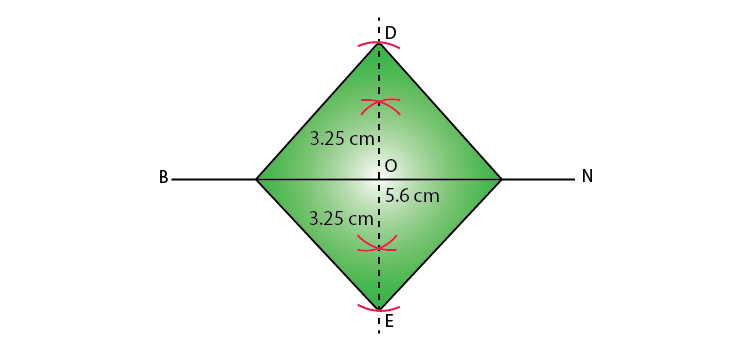BEND is the required Rhombus.

Q.4: Construct a Quadrilateral PLAN with PL = 4 cm LA = 6.5 cm ∠P = 90° ∠A = 110° ∠N = 85°.

Solution:

The sum of four angles of a quadrilateral is 360°.

∠P + ∠L + ∠A + ∠N = 360°

90° + ∠L + 110° + 85° = 360°

285° + ∠L = 360°

∠L = 360° − 285° = 75°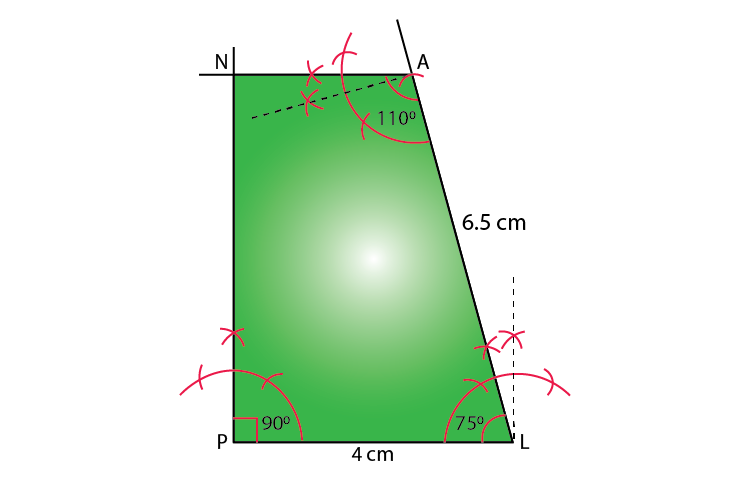Q.5: Construct a Parallelogram HEAR where HE = 5 cm EA = 6 cm ∠R = 85° .

Solution: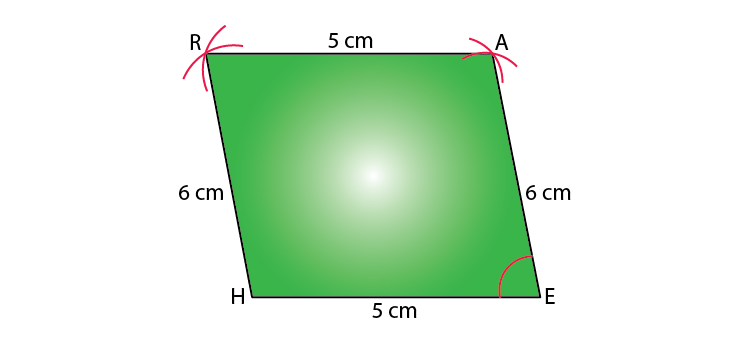Q.6: Construct a Quadrilateral DEAR where DE = 4 cm EA = 5 cm AR = 4.5 cm ∠E = 60°.

Solution:

Step 1: Draw a line segment DE of 4 cm and an angle of 60º at point E. As A is 5 cm away from E, cut the line segment EA 5 cm from point E on this ray.

Step 2: Again draw an angle of 90º at point A. As R is 4.5 cm away from A, cut a line segment RA of 4.5 cm from A on this ray.

Step 3: Join D to R.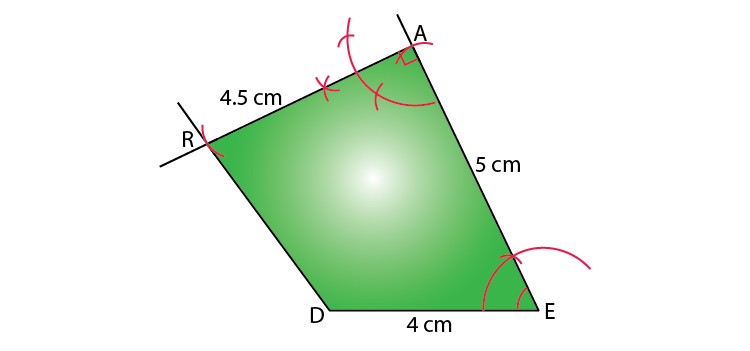Also check:

### Class 8 Chapter 4 -Practical Geometry Extra Questions

1. Construct a quadrilateral PQRS where PQ = 4.4 cm, QR = 4 cm, RS = 6.4 cm, SP = 3.8 cm and PR = 6.6 cm.
2. Construct a quadrilateral PQRS where PQ = 5.4 cm, QR = 2.5 cm, RS = 4 cm, SP =6.5 cm and PR = 5cm.
3. Construct a quadrilateral PQRS where PQ = 4 cm, QR = 5.6 cm, RS = 4.5 cm, SP = 5 cm AND PR = 6.5cm.
4. Construct a quadrilateral PQRS where PQ = 3.6 cm, QR = 5.5 cm, RS = 5 cm ∠B = 125° and ∠C= 80°.
5. Construct a quadrilateral PQRS where PQ = 6 cm, QR = 4 cm, RS = 4 cm, ∠Q = 95° and ∠R= 90°.
6. Construct a quadrilateral PQRS where PQ = 4 cm, QR = 3 cm, ∠P = 75° ∠Q = 80° and ∠R= 120°
7. Construct a quadrilateral PQRS where PQ = 4 cm, QR = 5 cm, ∠P = 50°,∠Q = 110° and ∠R= 70°.
8. Construct a quadrilateral PQRS where PQ = 6 cm, QR = 6 cm, RS = SP = 4.5 cm ∠Q = 120°
9. Construct a quadrilateral PQRS where PQ = 7.5 cm, QR = 6 cm, RS = 6 cm, SP =5 cm and PR = 10 cm.
10. Construct a quadrilateral PQRS where PQ = 5 cm, QR = 5.5 cm, RS = 2.5 cm, SP = 7.1 cm and PR = 8cm.
11. Construct the following Quadrilateral ABCD
AB = 4.5 cm
BC = 5.5 cm
CD = 4 cm